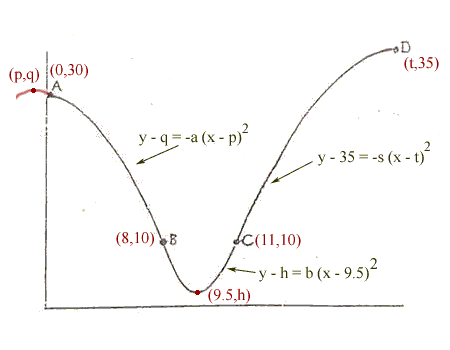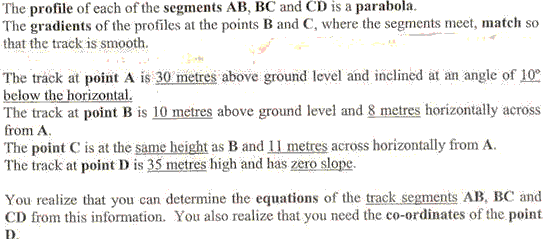Quandaries and Queries Dear Math Expert   I am a maths tutor.  One of my year 12 students has given me this assignment.  Many parts are ambiguous.  I am trying to determine the equation of the line segment AB.  Given that A has an incline of 10 degrees below the horizontal, I am assuming that this parabola (although you can only see part of it) has been rotated 10 degree clockwise.  Am I right in assuming this?  If so, how do I derive the equation of the parabola as it will then not fit the general form y = ax squared +bx +c of a parabola.    Also, how is one expected to find the x coordinate of D without the equation of this parabola.  To find the equation you need the x-coordinate and therefore be able to find another point on the parabola in order to derive the equation using simultaneous equations.    I am just blown away.  Please help. Hi, I labeled the diagram you sent with the given information, introducing variables where I needed them.The main part of the text you sent isIn your question you asked "Given that A has an incline of 10 degrees below the horizontal, I am assuming that this parabola (although you can only see part of it) has been rotated 10 degree clockwise.  Am I right in assuming this?" As you can see from the way I labeled the diagram, I assumed that the parabola that contains the segment AB has its vertex above and to the left of A at (p,q), and hence has equation y - q = -a (x - p)2. Since this parabola passes through A and B 30 - q = -a p2 and 10 - q = -a (8 - p)2 The slope of the tangent to this parabola at (0,30) is -tan(10o) so 2ap = -tan(10o) These three equations are enough to determine a, p and q, and thus the equation of the parabola. Can you complete the problem now? Penny Go to Math Central